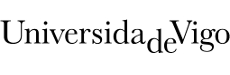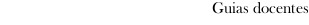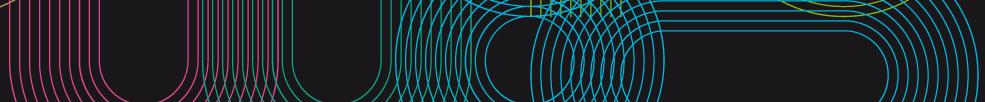Educational guide 2020_21Escola de Enxeñaría Aeronáutica e do Espazo
 Grado en Ingeniería AeroespacialSubjectsPhysics: Physics IContents
 Topic Sub-topic 1) Basic vectorial Calculus - Vectors and scalars - Coordinate system 2) Kinematics - Reference system, trajectories, velocity and acceleration - Rectilinear and curvilinear motion - Tangential and normal accelerations 3) Relative movement - Translation - Rotation - Components of the acceleration 4) Newton's laws - Force - Newtons first law  inertia - Newtons second law  weight - Newtons third law  action-reaction - Linear momentum - Angular momentum - Work and energy 5) Particle system - External and internal forces - Linear impulse. Collisions - Centre of mass. - Linear momentum, angular momentum, work and energy of a particle system 6) Rigid solids - Concept of rig solid. Centre of mass - Moment of inertia - Translation - Rotation around a fixed axis - Rolling motion 7) Particle statics and rigid solid statics - General equations of the equilibrium of rigid solid - System of forces - Stability 8) Fluid statics - Density and hydrostatic pressure - Archimedes principle - Surface tension. Capillarity
 Universidade de Vigo            | Reitoría | Campus Universitario | C.P. 36.310 Vigo (Pontevedra) | Spain | Tlf: +34 986 812 000